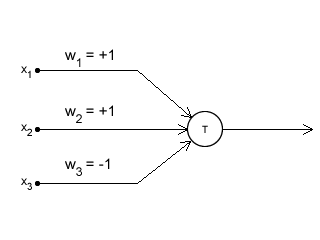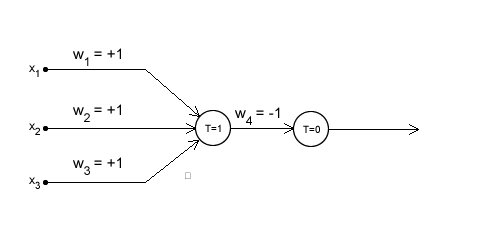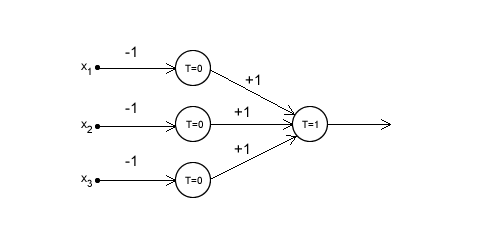## First artificial neurons: The McCulloch-Pitts model

The McCulloch-Pitts model was an extremely simple artificial neuron. The inputs could be either a zero or a one. And the output was a zero or a one. And each input could be either excitatory or inhibitory.

Now the whole point was to sum the inputs. If an input is one, and is excitatory in nature, it added one. If it was one, and was inhibitory, it subtracted one from the sum. This is done for all inputs, and a final sum is calculated.

Now, if this final sum is less than some value (which you decide, say T), then the output is zero. Otherwise, the output is a one.

Here is a graphical representation of the McCulloch-Pitts modelIn the figure, I represented things with named variables. The variables w1, w2 and w3 indicate which input is excitatory, and which one is inhibitory. These are called "weights". So, in this model, if a weight is 1, it is an excitatory input. If it is -1, it is an inhibitory input.

x1, x2, and x3 represent the inputs. There could be more (or less) inputs if required. And accordingly, there would be more 'w's to indicate if that particular input is excitatory or inhibitory.

Now, if you think about it, you can calculate the sum using the 'x's and 'w's... something like this:

sum = x1w1 + x2w2 + x3w3 + ...

This is what is called a 'weighted sum'.

Now that the sum has been calculated, we check if sum < T or not. If it is, then the output is made zero. Otherwise, it is made a one.

Now, using this simple neuron model, we can create some interesting things. Here are a few examples:

### NOR GateThe figure above is a 3 input NOR gate. A NOR gate gives you an output of 1 only when all inputs are zero (in this case, x1, x2 and x3) You can try the different possible cases of inputs (they can be either zero or one).

Note that this example uses two neurons. The first neurons receives the inputs you give. The second neuron works upon the output of the first neuron. It has no clue what the initial inputs were.

### NAND GateThis figure shows how to create a 3-input NAND gate with these neurons. A NAND gate gives a zero only when all inputs are 1. This neuron needs 4 neurons. The output of the first three is the input for the fourth neuron. If you try the different combinations of inputs.

The McCulloch-Pitts model is no longer used. These NOR and NAND gates already have extremely efficient circuits. So its pointless to redo the same thing, with less efficient models. The point is to use the "interconnections" and the advantages it has.

It has been replaced by more advanced neurons. The inputs can have decimal values. So can the weights. And the neurons actaully process the sum instead of just checking if it is less than or not.

#### Related posts#### 7 unique neural network architectures